Arduino

# How to Build a Simple Binary Clock With an Arduino and an LED MatrixFebruary 08, 2019 by Daniel Hertz
Share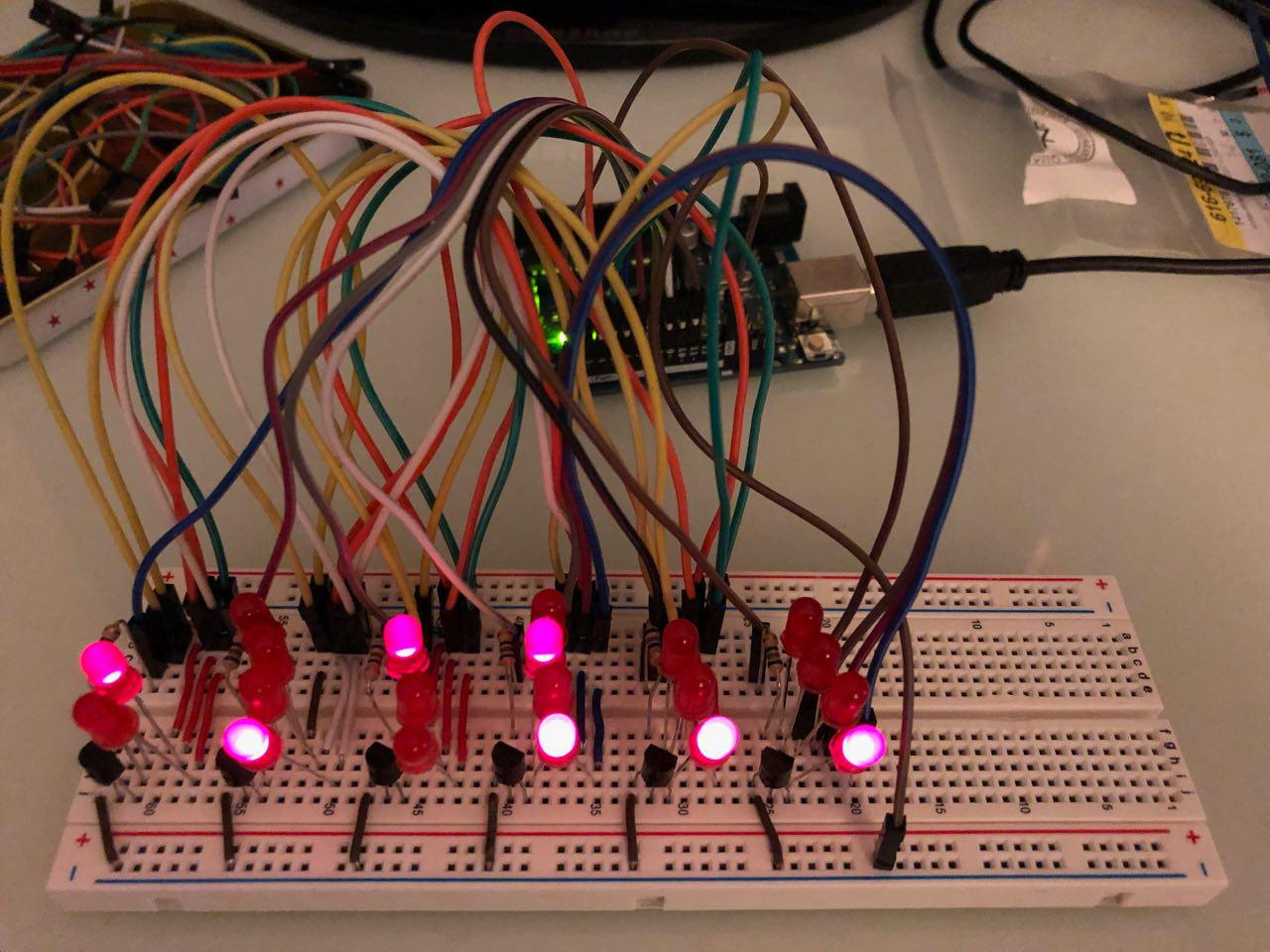In this project for beginners, we create a binary clock by building an LED matrix and using an Arduino.

## Hardware

 6 BC547 NPN transistor 20 LED (red) 4 150 ohm resistor 6 680 ohm resistor 1 Arduino Uno Rev. 3 1 Breadboard

This article will teach you about LED multiplexing and how to utilize this technique to build an array of LEDs that can be used in many different projects. In this particular case, weâ€™ll build a binary clock.

### How to Set up LED Multiplexing

This is a very simple technique for creating LED matrices where each individual diode can be addressed and turned on and off. Youâ€™ll first have to decide whether you want to build a common cathode or a common anode array. While the circuit is slightly different, both approaches do essentially work similarly: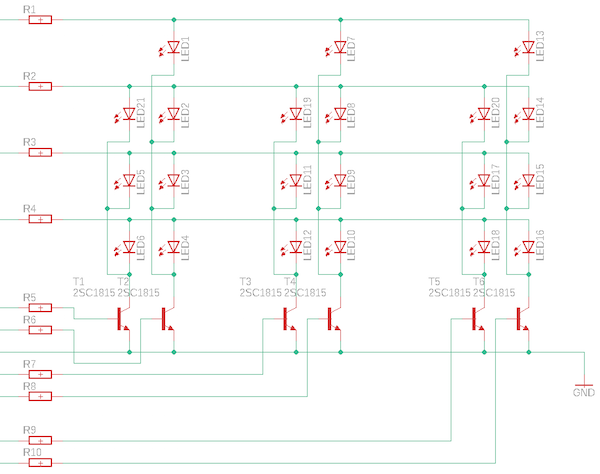The circuit diagram to create an LED matrix.

As you can see, weâ€™re building a common cathode matrix for this project. Therefore, the cathodes of the LEDs are linked together and then connected to GND via an NPN-transistor. The resistors R1 to R4 are the current limiting resistors for the LEDs and R5 to R10 are for the transistors.

### How to Address the Individual LEDs

Each diode in this array has its own address and can be turned on and off individually. If you have trouble addressing the LEDs, you can divide the array from the figure above into rows and columns and give each one a name like this: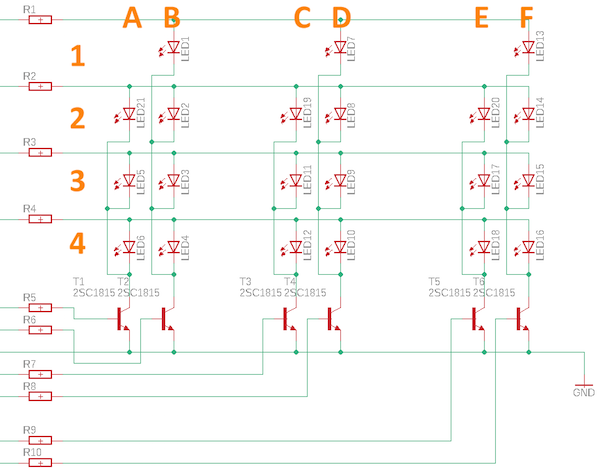Address each LED within the matrix by dividing the circuit into rows and columns.

To turn LED9 on, you need to supply a voltage at the base of transistor D and connect line 3 to the power supply. You can make it look like multiple LEDs are turned on by quickly switching between the one thatâ€™s currently active.

### Building the Binary Clock

Letâ€™s put our LED array to good use and build a binary clock using an Arduino Uno to turn the LEDs on and off, a DS3231, and a real-time clock module to keep track of the time.

Start by constructing the LED matrix from above. It looks a bit messy but all the jumper wires are needed to connect the LEDs: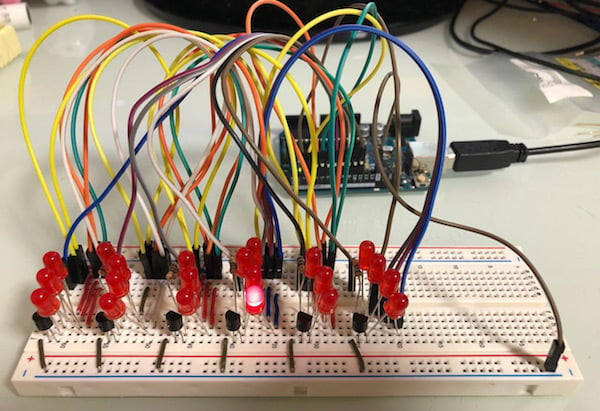Start by constructing your LED matrix.

Because this is a simple LED matrix, it uses ten I/O ports of the Arduino. So make sure to use one that has enough pins. If you want to reduce the number of necessary lines, you can use binary to decimal conversion. However, thatâ€™s beyond the scope of this article.

Rows 1 through 4 connect to the Arduino pins 2 through 5 and the 6 columns connect to pins 6 through 11. You can see these connections below.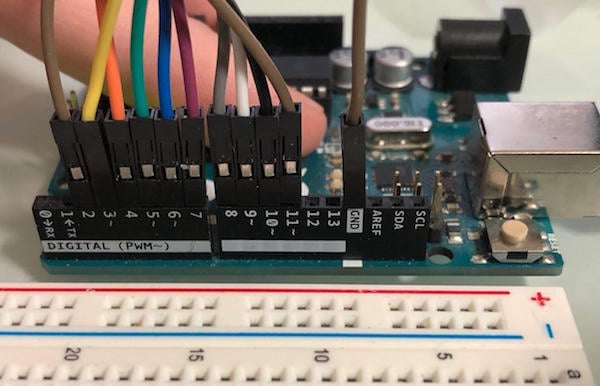Connect the LEDs to the Arduino.

### The Firmware

Start by writing a small test sketch that turns each LED in the matrix ON, one after the other. Your array is correctly wired if all of the LEDs light in the correct sequence.

``````void setup()
{
pinMode(2, OUTPUT);  // 1
pinMode(3, OUTPUT);  // 2
pinMode(4, OUTPUT);  // 3
pinMode(5, OUTPUT);  // 4
pinMode(6, OUTPUT);  // A
pinMode(7, OUTPUT);  // B
pinMode(8, OUTPUT);  // C
pinMode(9, OUTPUT);  // D
pinMode(10, OUTPUT); // E
pinMode(11, OUTPUT); // F
}
void loop()
{
for(int i = 6; i < 12; i++)
{
digitalWrite(i, 1);

for(int u = 2; u < 6; u++)
{
digitalWrite(u, 1);
delay(250);
digitalWrite(u, 0);
}

digitalWrite(i, 0);
}
}``````

As I mentioned above, the main firmware interfaces the DS3231 RTC module and reads the time. It then converts the values to binary and switches the corresponding LEDs ON.

Youâ€™ll need to download a library from Github for the Arduino IDE. The firmware is available as a downloadable .zip file at the end of this article. Once you upload the code, your Arduino should display the correct time â€” in this particular example, the time is 21:45:11.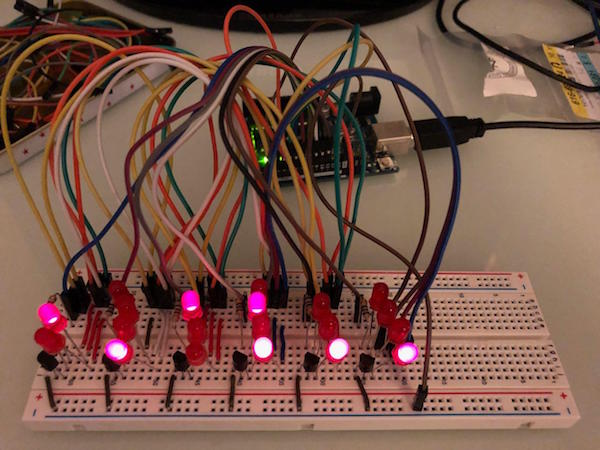Our completed binary clock.

### Why do Makers Use LED Matrices?

LED matrices can be quite useful in many applications, like this simple binary clock. Itâ€™s important to learn how transistors work as switches and how each LED in an array of components can be controlled individually.

However, this method is far from being perfect as it occupies many I/O ports and is limited to how many LEDs can be turned on simultaneously. But itâ€™s easy to understand and build.

If you want to reduce the number of necessary I/O lines, you can try using a BCD to Decimal decoder like the CD4028B (PDF), you can take a look at other techniques such as Charlieplexing, or you can use a completely different approach (e.g., addressable LED strips).